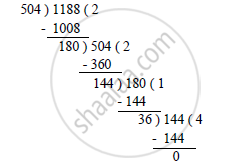# Using Euclid’S Algorithm, Find the Hcf Of 504 and 1188 . - Mathematics

Using Euclid’s algorithm, find the HCF of  504 and 1188 .

#### SolutionOn applying Euclid’s algorithm, i.e. dividing 1188 by 504, we get:
Quotient = 2, Remainder = 180
∴ 1188 = 504 × 2 + 180
Again on applying Euclid’s algorithm, i.e. dividing 504 by 180, we get:
Quotient = 2, Remainder = 144
∴ 504 = 180 × 2 + 144
Again on applying Euclid’s algorithm, i.e. dividing 180 by 144, we get:
Quotient = 1, Remainder = 36
∴ 180 = 144 × 1 + 36
Again on applying Euclid’s algorithm, i.e. dividing 144 by 36, we get:
∴ 144 = 36 × 4 + 0
Hence, the HCF of 1188 and 504 is 36.

Concept: Euclid’s Division Lemma
Is there an error in this question or solution?